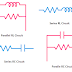Here in this article, we are going to know the uses, applications, and practical real-life examples of RL, RC, LC, and RLC Circuits. Before going to examples first of all we must know about these circuits. The 'RL' where R indicates the resistance and the L indicates the Inductance, so the RL circuit has both resistive and inductive elements either connected in series or in parallel. The 'RC' where R indicates the resistance and C indicates the Capacitance, so the RC circuit has both Resistive and Capacitive elements either connected in series or parallel. Similarly, 'LC' where L indicates the Inductance and C indicates the Capacitance, so the LC circuit has both Inductive and Capacitive elements either connected in series or parallel. The RLC circuit has all the resistive, inductive, and capacitive elements.

## RL Circuit Examples

The practical examples of RL Circuits are,
1. Ceiling Fan with Resistive Regulator Circuit - Yes, we know that the ceiling fan is built with an electric motor that is an inductive load or inductive element and the old resistive fan regulator is a resistive element. It can be called as a series RL circuit because the fan regulator is connected in series with the inductive coil of the fan.
2. Capacitance Compensator Circuit - An inductor is connected in parallel with the load resistor of any amplifier or processing circuit to compensate for the capacitance loading effect.

## RL Circuit Uses and Applications

RL circuits are used in,
1. Filter Circuits
2. Resonant Circuits
3. Signal Processing Circuits
4. Tuning Circuits
5. Current and voltage magnification circuits
6. Oscillator circuits

## RC Circuit Examples

The practical Examples of RC Circuit are,
1. Flash Light Circuit - We know that flashlight is built with a light and capacitor. The charging and discharging of the capacitor help for flashing. Here the light is a resistive element and the capacitor is the capacitive element.
2. Timer Circuit - Where a variable resistor is used with the capacitor. Using the variable resistor we can control the charging and discharging time of the capacitor so timing also can be controlled.
3. Relaxation Oscillator Circuit

## RC Circuit Uses and Applications

RC circuits are used in,
1. High-pass or Low Pass Filter Circuits
2. Traffic Light Control Circuits
3. Heart Pacemaker Circuits
4. Audio Equipment
5. An electronic integrator or differentiator circuits

## LC Circuit Examples

Some practical examples of LC circuits are,
1. LC Tank Circuit - It is constructed by connecting an inductor in parallel with a capacitor. Its main function of it is to generate the oscillating signal.
2. Capacitor Bank with Motor - Capacitor bank a capacitive element is connected in parallel with the motor(an inductive load) to improve the power factor.

## LC Circuit Uses and Applications

LC Circuits are used in,
2. Tuning Circuits
3. Audio Equilizer Circuits
4. Bandwidth filter circuits
5. Induction Heaters
6. RF Amplifiers

## RLC Circuit Examples

The practical examples of RLC Circuits are,
1. Oscillator Circuits - Here, inductive and capacitive elements are used to create oscillation whereas the resistive element is used to vary the time and other parameters.
2. Tuning Circuit - Here, also all the resistor, inductor, and capacitor is used.

## RLC Circuit Uses and Applications

RLC circuits are used in,
1. Audio Equipment
2. Filter Circuits
3. Resonance Circuits
4. Voltage and Current Magnification Circuits
5. Electronic Communication Devices

Applications and Examples of RL, RC, LC, RLC CircuitsReviewed by Author on 7:00 am Rating: 5